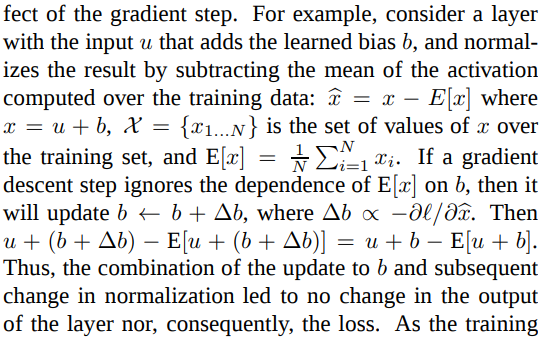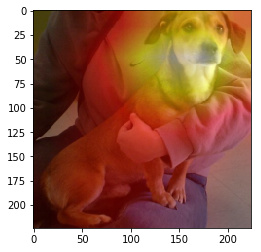# Wiki: Lesson 7

Can someone explain it at https://youtu.be/H3g26EVADgY?t=5340
Why SGD will undo the normalization while BatchNorm works?

@jeremy Could you elaborate that part a bit. After reading some articles, I get the intuition that why adding extra parameters could help in BatchNorm. But I still couldn’t get what do you mean when you are saying

1. SGD will undo it

You can try to read the original BatchNorm paper, it’s quite accessible. In section 2, they give a little example of a layer that adds a learned bias, and then centers the result (that is, subtracts the mean). It turns out that if you write the expression for the layer output after the gradient update, the bias update term cancels out. So, even when the optimization procedure changes the bias parameter, the update doesn’t change neither the layer output nor the loss.

It is not shown explicitly, but they claim that the same thing happens if you scale the input.

Here is a relevant excerpt from the paper (sorry for posting it as an image, but I can’t find how to typeset math in Discourse):1 Like

Thx, I actually just printed the paper out, will have a look soon.

Note that you need to use accuracy_np(preds,y) rather than accuracy(preds,y)

1 Like

Unless something change, during the January 2018 session I runned it with accuracy() function and all worked perfectly.
Even if accuracy_np() function works too, I think the sole difference is that accuracy() is the torch version and accuracy_np() is a numpy version of accuracy; please look at the function here :

Given the things, as the fastai library continuously evolves (new improvements), there is also a new cpu only version I will try later, if I get a bit of free time.

1 Like

Here’s a go from my level of understanding… Lets say SGD wants to push an activation function up, i.e. increase the mean (but here maintain the shape / variance). BN would reduce it, SGD would push up - but there’s no easy way for it to do so, so it would try changing lots of weights. BN would reduce it. = fail.
Now - all the previous weights stay the same, SGD changes the 1 scaling procedure and up it goes SGD happy, BN happy.
you can do a similar thought experiment for the m multiplier

How can I draw the CAM plot for the Dog class? I’m confused where the Cat class is specified in https://github.com/fastai/fastai/blob/master/courses/dl1/lesson7-CAM.ipynb

Hello,

I have a question regarding the stateful RNNs, and the behaviour of the hidden state during training and inference.

Training phase
Within a given chunk of text (one of the 64 pieces of text if batch size = 64 for example), assuming bptt = 8 as in the notebook example.

1. Batch 0. We take Characters 0 to 7 to predict characters 1 to 8. The hidden state at the end of batch 0 corresponds to the hidden state value to predict character 8 (result of the RNN time iteration at character 7).
2. Batch 1. We take Characters 8 to 15 to predict characters 9 to 16. For the first time iteration, we have the hidden state corresponding to the previous prediction at step 7, which contains the history of steps 0 to 6. Therefore this allows making stateful predictions with a hidden state taking the old values into account and the new and old positions match for the hidden state.

Inference phase
Reading at the code at the end of the notebook, the last cell generates a 400 characters prediction. The text is built character by character as follows:

1. Use characters 0 to 7 to predict character 8. Hidden state corresponds to character 7.
2. Use characters 1 to 8 to predict character 9. For the first character we take a hidden state “representing” the history at character 7 when we start the loop and make predictions for character 2.
3. Use characters 2 to 9 to predict character 10. For the first character we take a hidden state “representing” the history at character 8 to make predictions for character 3.

Basically I feel like the hidden state we should use as we loop through is not the hidden state at the end of a BPTT sequence, but the hidden state at the second iteration of the RNN loop.

Did I miss something?

hi, just started to watch the videos
when talking about the same thing, it’s described as
48:50 - neural net one hidden layer
49:25 - neural net no hidden layer
thanks

`m = CharSeqStatefulRnn(md.nt, n_fac, 512).cuda()`

We pass in 512 as batch size, but it should be 64, right? (since we set `bs=64` and pass it in as a param for `LanguageModelData.from_text_files`). I logged out the size of `self.h` and after the first iteration the dimension corrects to `[1, 64, 256]`, since we check if `self.h.size(1) != bs`, but I was a bit confused where 512 came from.

Have written a blog on Generating your own music using RNNs. Hope you enjoy it.

https://goo.gl/5h9UJM

5 Likes

Has anyone else tried to get the CAM things at the end working on specific input images. Can get an prediction out for test image (using learn.predict_array) but struggling to work out how to get heatmap to see different parts of images for individual test image.

@jeremy I have a doubt regarding resnet.
The resnet block simply does the operation: x + f(x) where x is the input and f(x) is output of BnLayer. But the dimensions of input and output won’t be same so how can these two be added?

Below line will make sure that batch size is set according to batch passed from training_data_loader.
if self.h.size(1) != bs: self.init_hidden(bs)

Hi Shivam,
I understand you are referring overridden “forward” method of “ResnetLayer” class.

Please note that we are passing stride=1 while instantiating “ResnetLayer” class,hence it will create conv2d object with 1 stride during class instantiation.(check init of “BnLayer” class)

self.layers2 = nn.ModuleList([ResnetLayer(layers[i+1], layers[i + 1], 1) for i in range(len(layers) - 1)])

When you call f(x) i.e. super.forward() ,forward method passes input x to conv object where stride is 1 hence it does not half the input after convolution and dimensions remain unchanged.

Hope it helps.It also works for dogs, you just need to find one in your validation set.

a=iter(data.val_dl)
for i in range(20): #dog 20 in my validation set
x,y = next(a)
x,y = x[None,1], y[None,1]

sfs = [SaveFeatures(o) for o in [m[-7], m[-6], m[-5], m[-4]]]
py = m(vx)
py = np.exp(to_np(py)) # size 2 (1,0) cat (0,1) dog
print (‘Cat’) if py==1 else print (‘Dog’)

for o in sfs: o.remove()
feat = np.maximum(0,to_np(sfs.features)) #values coming out of the final convolutional layer (2,7,7)
f2=np.dot(np.rollaxis(feat,0,3), py) # catness or dogness
f2-=f2.min()
f2/=f2.max() #probabilites of catness or dogness

dx = data.val_ds.denorm(x)
plt.imshow(dx)
plt.imshow(scipy.misc.imresize(f2, dx.shape), alpha=0.5, cmap=‘hot’);

Hi, I’m new here and I have a question about the code of GRUCell in Lesson 7.

Jeremy’s reference code is as follows:

``````def GRUCell(input, hidden, w_ih, w_hh, b_ih, b_hh):
gi = F.linear(input, w_ih, b_ih)
gh = F.linear(hidden, w_hh, b_hh)
i_r, i_i, i_n = gi.chunk(3, 1)
h_r, h_i, h_n = gh.chunk(3, 1)

resetgate = F.sigmoid(i_r + h_r)
inputgate = F.sigmoid(i_i + h_i)
newgate = F.tanh(i_n + resetgate * h_n)
return newgate + inputgate * (hidden - newgate)
``````

I felt confused by the last line `newgate + inputgate * (hidden - newgate)` , which corresponds to equation

h_t = (1-z_t) * h_{t-1} + z_t * h_t

It is seemingly supposed to be written (in a similar format) as
`hidden + inputgate * (newgate - hidden)`.

So is there something wrong with this understanding?
@jeremy Hope this “call” not be a bother…

I’m having some difficulties understanding what’s going on in the CAM section. Specifically trying to replicate processing a model layer. In the notebook, these cells run fine:

``````sfs = [SaveFeatures(o) for o in [m[-7], m[-6], m[-5], m[-4]]]
for o in sfs: o.remove()
[o.features.size() for o in sfs]
``````

Where the final line shows the sizes of the items in sfs.

However, when I try to replicate this on a single layer, I get an error.

``````test = [SaveFeatures(o) for o in [m[-4]]]
for o in test: o.remove()
[o.features.size() for o in test]
``````

Raises

``````AttributeError: 'NoneType' object has no attribute 'size'
``````

I can try both methods in the same notebook and the first will work while the second will not. My intuition is that this is SaveFeatures returning

``````features=None
``````

But I’m not sure what to do about it. Is there something happening in SaveFeatures that I’m missing?

You need to call the model after SaveFeature and then view feature size

``````test = [SaveFeatures(o) for o in [m[-4]]]
py = m(Variable(x.cuda()))
for o in test: o.remove()
[o.features.size() for o in test]``````